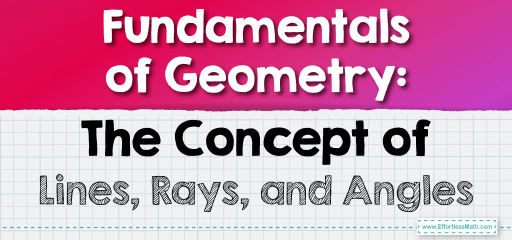# Fundamentals of Geometry: The Concept of Lines, Rays, and Angles

In the study of geometry, three fundamental concepts are lines, rays, and angles. A line extends infinitely in two directions, a ray has a starting point and extends infinitely in one direction, and an angle measures the rotation between two intersecting lines or rays. Together, these basic elements form the building blocks for more advanced geometric topics. This guide simplifies and introduces these foundational concepts for easy comprehension.## Step-by-step Guide: Lines, Rays, and Angles

Lines:
A line is an infinite set of points stretching endlessly in both directions. Imagine a road with no beginning or end – that’s our geometric line.
Representation: A line segment, with two defined endpoints, is usually drawn to depict a line. If we use points $$A$$ and $$B$$ to indicate the segment’s ends, the line is represented as $$\overleftrightarrow{AB}$$.

Rays:
Think of a ray as half of a line. It starts at a point and travels infinitely in just one direction. It resembles a beam of light originating from its source and illuminating indefinitely ahead.
Representation: With $$A$$ being the starting point and $$B$$ a point indicating its direction, the ray can be denoted as $$\overrightarrow{AB}$$.

Angles:
An angle forms when two rays share a starting point. Visualize it as the opening between two doors originating from the same hinge. The degree of rotation connecting one ray with the other is the angle’s measurement.
Measurement: We measure angles in degrees using a protractor. The shared starting point is the vertex, and the rays are the angle’s arms. If $$A$$ is the starting point with rays extending through $$B$$ and $$C$$, we represent the angle as $$\angle BAC$$ or $$\angle CAB$$.

### Examples

Example 1: Identifying Geometric Elements
A drawing features point $$A$$ stretching infinitely towards points $$B$$ and $$C$$ in opposite directions. Another ray commences from point $$D$$ and extends unendingly towards point $$E$$. These rays intersect at $$D$$. Solution:
We have:

• Line $$\overleftrightarrow{BC}$$ traversing through point $$A$$.
• Ray $$\overrightarrow{DE}$$ originating at point $$D$$.
• Angle $$\angle BDA$$ materializing from the rays’ intersection.

Example 2: Measuring an Angle
A depicted angle created by rays sharing point $$O$$ and stretching out through points $$P$$ and $$Q$$.

Solution:
To measure $$\angle POQ$$, place the protractor’s midpoint on $$O$$. Ensure the base aligns with $$\overrightarrow{OP}$$. The intersect of $$\overrightarrow{OQ}$$ with the protractor provides the angle’s measurement.

### Practice Questions:

1. When two rays originate from an identical point but traverse opposite directions, which angle do they form?
2. Employing a real-world analogy, differentiate between a line and a ray.
3. Sketch an angle and then bisect it using only a compass and straightedge.

1. Such rays constitute a straight angle measuring $$180^\circ$$.
2. Visualize a line as a two-way endless road, while a ray resembles a one-way street beginning at a certain point and continuing endlessly.
3. (Illustrative solution: After creating an angle, use the compass to draft an arc intersecting both of the angle’s sides. Without adjusting the compass width, position it on the intersecting points to craft two more arcs inside the angle. The bisector of the angle is where these arcs converge.)

### What people say about "Fundamentals of Geometry: The Concept of Lines, Rays, and Angles - Effortless Math: We Help Students Learn to LOVE Mathematics"?

No one replied yet.

X
30% OFF

Limited time only!

Save Over 30%

SAVE $5 It was$16.99 now it is \$11.99